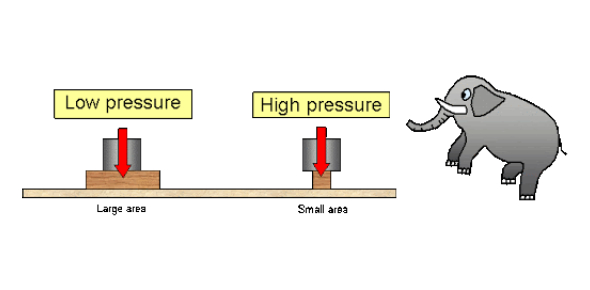# Pressure Quiz: Ultimate Questions!

10 Questions | Total Attempts: 3610Settings.

• 1.
Which of the following is the definition of pressure?
• A.

Pressure is the force acting on an object in a downwards direction.

• B.

Pressure is the force per unit area applied in a direction parallel to the surface.

• C.

Pressure is the force per unit area applied in a direction perpendicular to the surface.

• D.

Pressure is the force per unit volume applied in a direction perpendicular to the surface.

• 2.
Which of the following is the unit for Pressure? I. Pa II. Nm III. N/m2
• A.

I only

• B.

III only

• C.

I and III only

• D.

II and III only

• 3.
The figure below shows a box of mass 2 kg. What is the weight of the box on Earth?
• A.

0.2 N

• B.

2 N

• C.

20 N

• D.

200 N

• 4.
What is the minimum pressure that the box in Q3 can exert on the floor?
• A.

26.7 Pa

• B.

267 Pa

• C.

667 Pa

• D.

2220 Pa

• 5.
What is the maximum pressure that the box in Q3 can exert on the floor?
• A.

26.7 Pa

• B.

267 Pa

• C.

667 Pa

• D.

2220 Pa

• 6.
The diagram below shows a simple hydraulic system use to lift up a load. Given that the area of piston A is 1 cm2 (0.0001 m2), calculate the pressure exerted on piston A.   Uploader, S.Calculating the mechanical advantage of a hydraulic system, Connexions Web site. http://cnx.org/content/m31723/1.1/, Aug 27, 2009.
• A.

50 Pa

• B.

500 Pa

• C.

5000 Pa

• D.

500000 Pa

• 7.
Since liquid cannot be compressed, the pressure exerted on piston A will be transmitted to piston B.  What is the load that can be lifted up at piston B?
• A.

0.5 N

• B.

50 N

• C.

500 N

• D.

5000 N

• 8.
Which of the following statements is correct?
• A.

In the design of a dam, the thickness of the wall is the same as the pressure of water remains the same.

• B.

In the design of a dam, the wall at the bottom of the dam is thicker as the pressure of water increases with depth.

• C.

In the design of a dam, the wall at the bottom of the dam is thinner as the pressure of water decreases with depth.

• D.

In the design of a dam, the wall at the middle of the dam is thicker as the pressure of water is highest in the middle.

• 9.
The image shows a cross-sectional view of an air foil moving through air. Which of the following statements is correct?
• A.

The speed of the air moving at the upper surface of the air foil is less than the speed of the air moving at the lower surface of the air foil.

• B.

The speed of the air moving at the upper surface of the air foil is more than the speed of the air moving at the lower surface of the air foil.

• C.

The speed of the air moving at the upper surface of the air foil is the same as the speed of the air moving at the lower surface of the air foil.

• D.

There is not sufficient information to make a conclusion.

• 10.
The image shows a cross-sectional view of an airfoil moving through air. Which of the following statements describe the motion of the air foil in the vertical direction.
• A.

The air foil is moving downwards with increasing speed.

• B.

The air foil is moving upwards at a constant speed.

• C.

The air foil is moving upwards with decreasing speed.

• D.

The air foil is moving upwards with increasing speed.

Related TopicsBack to top